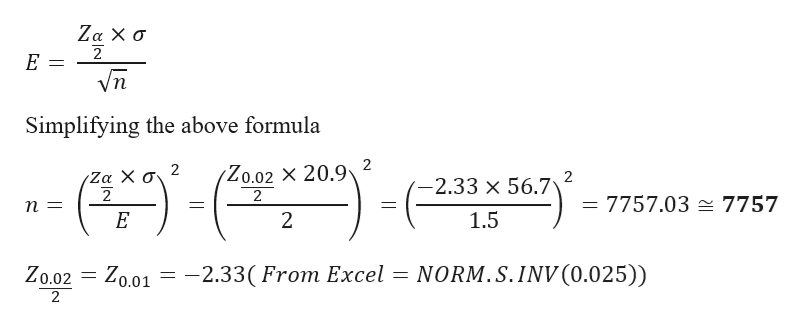# You want to obtain a sample to estimate a population mean. Based on previous evidence, you believe the population standard deviation is approximately σ=56.7σ=56.7. You would like to be 98% confident that your estimate is within 1.5 of the true population mean. How large of a sample size is required? Round up to nearest whole number.n =

Question
6 views

You want to obtain a sample to estimate a population mean. Based on previous evidence, you believe the population standard deviation is approximately σ=56.7σ=56.7. You would like to be 98% confident that your estimate is within 1.5 of the true population mean. How large of a sample size is required? Round up to nearest whole number.

n =

check_circle

Step 1

Margin of error, Sample size

Given data

Standard deviation = 56.7

Confidence interval = 0.98

Significance level(α) = 1- 0....help_outlineImage TranscriptioncloseΖα Χ σ E = Vn Simplifying the above formula 2 (Zo.02 - 20.9 2 Ζα Χ σ 2 -2.33 x 56.7 2 = 7757.03 7757 1.5 E 2 -2.330 From Excel = NORM.S.INV(0.025)) Zo.02 Zo.01 2 fullscreen

### Want to see the full answer?

See Solution

#### Want to see this answer and more?

Solutions are written by subject experts who are available 24/7. Questions are typically answered within 1 hour.*

See Solution
*Response times may vary by subject and question.
Tagged in

### Hypothesis Testing